International
Tables for
Crystallography
Volume A
Space-group symmetry
Edited by M. I. Aroyo

International Tables for Crystallography (2015). Vol. A, ch. 1.4, pp. 42-43

## Section 1.4.1.3. Schoenflies symbols

H. Wondratscheke

#### 1.4.1.3. Schoenflies symbols

| top | pdf |

The Schoenflies symbols were introduced by Schoenflies (1891, 1923). They describe the point-group type, also known as the geometric crystal class or (for short) crystal class (cf. Section 1.3.4.2), of the space group geometrically. The different space-group types within the same crystal class are denoted by a superscript index appended to the point-group symbol.

#### 1.4.1.3.1. Schoenflies symbols of the crystal classes

| top | pdf |

Schoenflies derived the point groups as groups of crystallographic symmetry operations, but described these crystallographic point groups geometrically by their representation through axes of rotation or rotoreflection and reflection planes (also called mirror planes), i.e. by geometric elements; for geometric elements of symmetry elements, cf. Section 1.2.3, de Wolff et al. (1989, 1992) and Flack et al. (2000). Rotation axes dominate the description and planes of reflection are added when necessary. Rotoreflection axes are also indicated when necessary. The orientation of a reflection plane, whether horizontal, vertical or diagonal, refers to the plane itself, not to its normal.

A coordinate basis may be chosen by the user: the basis vectors start at the origin which is placed in front of the user. The basis vector c points vertically upwards, the basis vectors a and b lie more or less horizontal; the basis vector a pointing at the user, b pointing to the user's right-hand side, i.e. the basis vectors a, b and c form a right-handed set. Such a basis will be called a conventional crystallographic basis in this chapter. (In the usual basis of mathematics and physics the basis vector a points to the right-hand side and b points away from the user.) The lengths of the basis vectors, the inclination of the ab plane relative to the c axis and the angles between the basis vectors are determined by the symmetry of the point group and the specific values of the lattice parameters of the crystal structure.

The letter C is used for cyclic groups of rotations around a rotation axis which is conventionally c. The order n of the rotation is appended as a subscript index: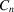; Fig. 1.4.1.1(a) represents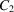. The values of n that are possible in the rotation symmetry of a crystal are 1, 2, 3, 4 and 6 (cf. Section 1.3.3.1for a discussion of this basic result). The axis of an n-fold rotoreflection, i.e. an n-fold rotation followed or preceded by a reflection through a plane perpendicular to the rotation axis (such that neither the rotation nor the reflection is in general a symmetry operation) is designated by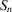, see Fig. 1.4.1.1(b) for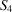.Figure 1.4.1.1 | top | pdf |Symmetry-element diagrams of some point groups [adapted from Vainshtein (1994)]. The point groups are specified by their Schoenflies and Hermann–Mauguin symbols. (a) C2 = 2, (b)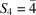, (c) D3 = 32, (d) C4h = 4/m, (e) D6h = 6/m2/m2/m, (f) C3v = 3m, (g)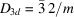, (h) T = 23. [The cubic frame in part (h) has no crystallographic meaning: it has been included to aid visualization of the orientation of the symmetry elements.]

The following types of point groups exist:

• (1) cyclic groups

 (a) of rotations (C):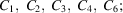(b) of rotoreflections (S, for the names in parentheses see later):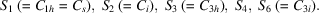• (2) In dihedral groups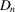an n-fold (vertical) rotation axis is accompanied by n symmetry-equivalent horizontal twofold rotation axes. The symbols are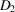[in older literature, as in IT (1952), one also finds V instead of D2, taken from the Vierergruppe of Klein (1884)],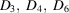;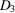is visualized in Fig. 1.4.1.1(c).

• (3) Other crystallographic point groups can be constructed by arotation axis or acombination of rotation axes with a horizontal symmetry plane, leading to symbolsor: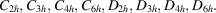The point groupsandare represented by Figs. 1.4.1.1(d) and 1.4.1.1(e).

• (4) Vertical rotation axescan be combined with a vertical reflection plane, leading to n symmetry-equivalent vertical reflection planes (denoted v) which all contain the rotation axis: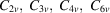with Fig. 1.4.1.1(f) for.

• (5) Combinationsof rotation axes may be combined with vertical reflection planes which bisect the angles between the horizontal twofold axes, such that the vertical planes (designated by the index d for `diagonal') alternate with the horizontal twofold axes: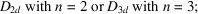see Fig. 1.4.1.1(g) for. In both point groups rotoreflections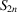, i.e.or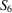, occur. Note that the classification of crystal classes into crystal systems follows the order of rotoinversions, not that of rotoreflections(cf. Section 1.2.1for the definition of rotoinversions). Therefore,is tetragonal (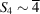) andis trigonal because of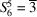). Analogously,andare hexagonal because they contain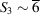. The point groups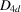and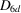are not crystallographic as they contain noncrystallographic eightfold or 12-fold rotoreflections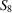or.

• (6) In all these groups the directions of the vectors ±c are not equivalent to any other directions. There are, however, also cubic point groups and thus cubic space groups in which the basis vector c is symmetry-equivalent to both basis vectors a and b. T,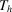and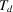can be derived from the rotation group T of the tetrahedron, see Fig. 1.4.1.1(h). O and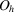can be derived from the rotation group O of the octahedron. The indices h and d have the same meaning as before.

• (7) Some of these symbols are no longer used but are replaced by more visual ones.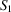describes a reflection through a horizontal plane, it is replaced now by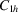or by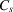;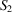describes an inversion in a centre, it is replaced by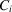. The symboldescribes the same arrangement asand is thus not used.contains an inversion centre combined with a threefold rotation axis and is replaced by.

The description of crystal classes using Schoenflies symbols is intuitive and much more graphic than that by Hermann–Mauguin symbols. It is useful for morphological studies investigating the symmetry of the ideal shape of crystals. Schoenflies symbols of crystal classes are also still used traditionally by physicists and chemists, in particular in spectroscopy and quantum chemistry.

#### 1.4.1.3.2. Schoenflies symbols of the space groups

| top | pdf |

Different space groups of the same crystal class are distinguished by their superscript index, for example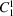;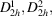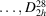or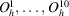.

Schoenflies symbols display the space-group symmetry only partly. Therefore, they are nowadays rarely used for the description of the symmetry of crystal structures. In comparison with the Schoenflies symbols, the Hermann–Mauguin symbols are more indicative of the space-group symmetry and that of the crystal structures.

### References

International Tables for X-ray Crystallography (1952). Vol. I, Symmetry Groups, edited by N. F. M. Henry & K. Lonsdale. Birmingham: Kynoch Press. [Abbreviated as IT (1952).]
Flack, H. D., Wondratschek, H., Hahn, Th. & Abrahams, S. C. (2000). Symmetry elements in space groups and point groups. Addenda to two IUCr reports on the nomenclature of symmetry. Acta Cryst. A56, 96–98.
Klein, F. (1884). Vorlesungen über das Ikosaeder. Leipzig: Teubner.
Schoenflies, A. (1891). Krystallsysteme und Krystallstruktur. Leipzig: B. G. Teubner. [Reprint (1984). Berlin: Springer-Verlag.]
Schoenflies, A. (1923). Theorie der Kristallstruktur. Berlin: Gebrüder Borntraeger.
Wolff, P. M. de, Billiet, Y., Donnay, J. D. H., Fischer, W., Galiulin, R. B., Glazer, A. M., Hahn, Th., Senechal, M., Shoemaker, D. P., Wondratschek, H., Wilson, A. J. C. & Abrahams, S. C. (1992). Symbols for symmetry elements and symmetry operations. Final report of the International Union of Crystallography Ad-Hoc Committee on the Nomenclature of Symmetry. Acta Cryst. A48, 727–732.
Wolff, P. M. de, Billiet, Y., Donnay, J. D. H., Fischer, W., Galiulin, R. B., Glazer, A. M., Senechal, M., Shoemaker, D. P., Wondratschek, H., Hahn, Th., Wilson, A. J. C. & Abrahams, S. C. (1989). Definition of symmetry elements in space groups and point groups. Report of the International Union of Crystallography Ad-Hoc Committee on the Nomenclature of Symmetry. Acta Cryst. A45, 494–499.